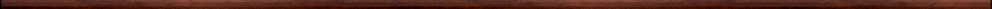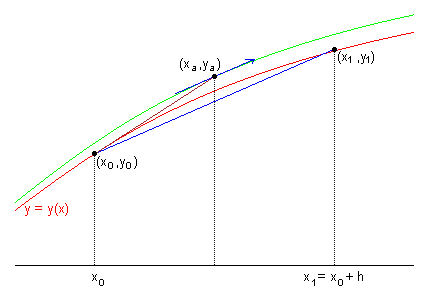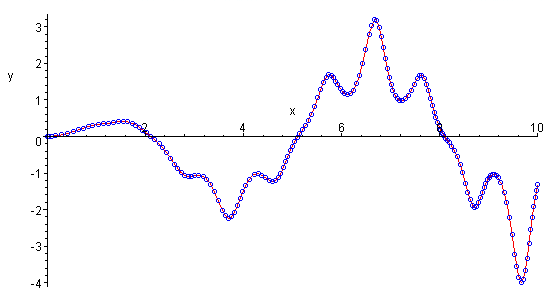Maple worksheets on the numerical solution of differential equationsNumerical methods topics:

They are all compatible with Classic Worksheet Maple 10.Introduction - DEintro.mws

• Using Maple's dsolve procedure to solve first order differential equations.
• Plotting solutions and drawing direction fields.

Euler's method - euler.mws

• What are numerical solutions for differential equations?
• Description of Euler's method.
• A procedure which can be used to implement Euler's method with a fixed step-size: desolveRK( . . ,method=euler).
The procedure desolveRK is designed to allow input parameters which are similar to those of dsolve. The output is a list of points which can be then easily be plotted.
A tabulated comparison with an analytical solution, when available, can be made using a utility routine: comparewithfcn.

Taylor series method - taylorDE.mws

• Description of the Taylor series method for first order differential equations.
• A procedure which can be used to implement the Taylor series method: desolveTA.
The output can be a list of points, or a procedure which uses local Taylor series to interpolate between the fixed points along the approximate solution curve.
An option to use adaptive step-size control is available, which constructs a more efficient and reliable local Taylor series approximation for the solution.

Runge-Kutta order 2 methods - rungk2.mws

• Description of the improved Euler method and the Runge-Kutta mid-point method.
• General order 2 methods.
• Derivation of the general order 2 Runge-Kutta scheme.
• The procedure desolveRK can be used to obtain discrete numerical solutions for 1st order differential equations using the Runge-Kutta methods with order from 2.
The options method=impeuler and method=rk2 can be used to implement the improved Euler and mid-point methods, and the option alpha=a, where a is between 0 and 1, can be used to implement a general order 2 Runge-Kutta method in which the second function evaluation is made at the fraction alpha across the step.

General Runge-Kutta methods - rungk.mws

• The classical order 3 and 4 Runge-Kutta methods.
• General order 3 Runge-Kutta methods and coefficient systems for some order 3 methods.
• General order 4 Runge-Kutta methods and coefficient systems for some order 4 methods.
• Description of the general Runge-Kutta scheme.
• The procedure desolveRK can be used to obtain discrete numerical solutions for 1st order differential equations using various Runge-Kutta methods with orders from 2 to 9.

• Description of adaptive step-size control for Runge-Kutta methods.
• The procedure desolveRK can be used as a general "work-horse" for solving first order differential equations numerically. 4-5, 5-6, 6-7, 7-8 and 8-9 order methods are available. There is an option to see how the the underlying discrete solution is constructed. The output of both methods is in the form of a procedure which can be evaluated throughout a predetermined interval.
In the default mode the interpolation is performed by means of a single Runge-Kutta step, which requires evaluations of the gradient field; however, in the case of the 4-5 method, there is an option for the output to be in the form of a procedure which performs interpolation through the storage of extra parameters so that no new evaluations of the gradient field are needed.
• Construction of a numerical solution in the form of a procedure using Maple's procedure dsolve, via the option type=numeric.

More Runge-Kutta examples - RKexamp.mws

Runge-Kutta examples related to RL and RC circuits - RKexamp2.mws

The pet rock problem - rock.mws

• Description of the problem
• Runge Kutta solution using dsolve
• Solution via a numerical inverse function
• Solution using desolveRK

Sensitivity of solutions for 1st order DE's to initial conditions - sensRK.mws

• Sensitivity of solutions to initial conditions
• Convergence and divergence of solutions

Checking the accuracy of a solution by "back-tracking" - backRK.mws

• Back-tracking to check the accuracy of a numerical solution for a DE
• Sensitivity problems in connection with back-tracking

Procedures for differential equations - DEsol.zipTop of page

Main index